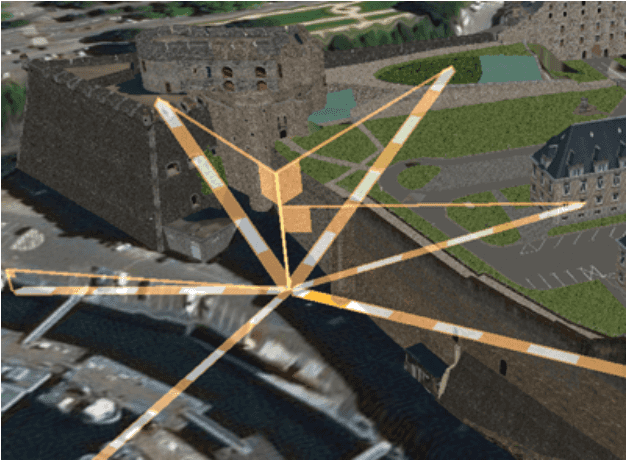# Make measurements

Just as there are many types of projections available to display the earth's surface on a two-dimensional map, there are a variety of methods for measuring distance or area. As when choosing a projection, you need to understand the characteristics of measurement methods when making measurements for a particular use.

The following are some questions to ask when working with geographic measurements:

• Will measurements be at a large or small scale? The curvature of the earth's surface is not as much of a factor for measurements made at a larger scale (smaller areas).

• What coordinate system will be used? With a projected coordinate system, the properties of the underlying projection greatly influence the accuracy of measurements in a given area of the map.

• How accurate do measurements need to be? Sometimes you want to interactively explore using approximate measurements. At other times, a high level of accuracy is required.

• Will measurements include things above or below the surface of the earth? Some measurement methods do not consider the z-value (elevation or altitude) for the geometry being measured.

• Should measurements take topography into account? Distance for a hiking trail, for example, is more accurate if it accounts for travel across the surface terrain.

• Are measurements being made for travel on a predefined line network (representing roads or trails, for example). See Route and directions to see how to model this kind of travel and make measurements in distance and time or other costs.

## Planar versus geodetic measurements

Planar measurements are made with straight lines in two dimensions. If you're using a geographic coordinate system, planar measurements must cut through the curved surface of the earth rather than following it (similar to pressing a ruler into a globe). This causes the distance measurement to be shorter than the true distance, and the error becomes greater as the length of the line increases.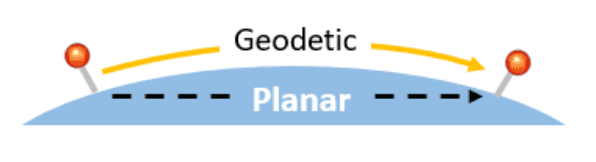Planar measurements using a projected coordinate system are like placing a ruler on a paper map. In this case, the properties of the underlying projection influence the accuracy of measurements in a given area. A Web Mercator projection, for example, exaggerates areas of the map that are not near the equator. Measurements will, therefore, be most accurate near the equator and error will increase as you move toward the poles. Planar measurements are generally best if made over small areas in a suitable spatial reference, such as the correct UTM zone for the area.

Measurements relative to a curved surface (nonplanar) are generically referred to as geodetic. Although not an exact representation of the earth, and measurements do not follow actual features on the surface (terrain, in other words), geodetic measurements are more accurate than planar measurements, especially over long distances.

For example, the following image shows distance measurements between Reykjavik and Saint Petersburg in a Web Mercator map. The red line is a planar measurement and the light gray line is geodetic. If a similar measurement were made near the equator, the values would be much closer.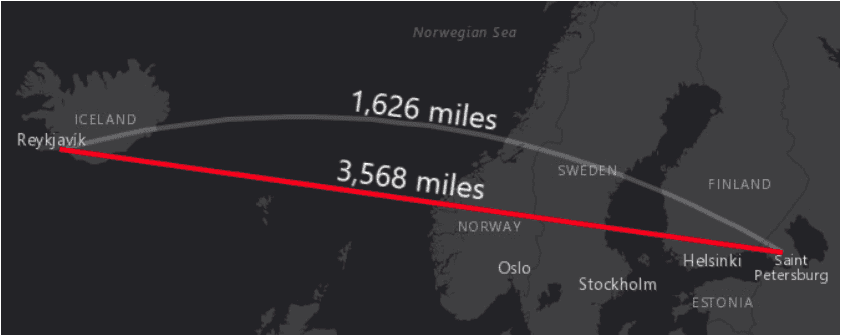The following are a variety of geodetic measurement types:

• Great circle—Any circle or near circle produced by the intersection of the surface of a sphere and a flat plane that passes through the center of the sphere. The equator and all lines of longitude are great circles. Great circles are used in navigation, since the shortest path between two points on the earth's surface lies on a great circle.

• Geodesic—The shortest distance between two points on the surface of a spheroid (also known as an ellipsoid). For example, the shortest path between two points along a meridian form a geodesic. This is similar to the great circle method, which models the surface as a sphere.

• Normal section—A normal section is a simplified version of a geodesic line. It's defined by the intersection of a plane that passes through two points on the surface of the spheroid and is perpendicular to the surface (normal) at the first point.

• Loxodrome—A line between two locations at a constant bearing is known as a loxodrome (or rhumb line). Loxodromes do not attempt to calculate the shortest distance since the line between the points is defined by the bearing. However, they have historical significance for nautical navigation, where a ship or plane could follow a constant compass bearing from one point to another. Only when a loxodrome is oriented precisely north-south or east-west does it define a geodesic (shortest path) line.

The geodetic methods described previously account for the earth's curvature, which makes them well-suited for nautical and aeronautical routes. However, they ignore surface topography and thus are not ideal for measuring land travel over terrain with a lot of relief. Surficial measurement, on the other hand, can be used to measure distance as it would be traveled along the terrain. With ArcGIS Pro and ArcGIS Desktop, for example, you can use the Add surface information tool to add length or area fields to a vector dataset whose values are calculated from an input elevation surface. Geoprocessing also provides a way to implement custom measurement tools.

Similarly, calculating routes along a transportation network is the most accurate way to measure travel (distance and time) over roads or trails. For information about modeling travel across a network, see Route and directions.

## Use the geometry engine to make measurements

`GeometryEngine` provides several methods for making planar or geodetic measurements of distance or area. These measurements are made independent of the display (no map or scene is necessary). Planar measurements use the spatial reference and units of the input geometry. Even if in a geographic coordinate system, planar measurements do not consider the curvature of the earth and will return values in decimal degrees. You should therefore project the geometry you want to measure into an appropriate projected coordinate system before making planar measurements.

The geometry engine methods `area()`, `GeometryEngine.distanceBetween()`, and `length()` calculate planar measurements. They have the geodetic equivalents `areaGeodetic()`, `GeometryEngine.distanceGeodetic()`, and `lengthGeodetic()`. If a geometry engine method doesn't contain the word geodetic, you can assume it's a planar operation.

The following code makes a planar distance measurement between Edinburgh and Dar es Salaam. The input locations are first projected to a world equidistant projection.

Use dark colors for code blocksCopy

``````// Define geographic map points for the cities of Edinburgh (Scotland) and Dar es Salaam (Tanzania).
edinburghGeographic = new Point(-3.1883, 55.9533, 0.0, SpatialReferences.getWgs84());
darEsSalaamGeographic = new Point(39.2083, -6.7924, 0.0, SpatialReferences.getWgs84());

// Create a world equidistant cylindrical spatial reference for measuring planar distance.
SpatialReference equidistantSpatialRef = SpatialReference.create(54002);

// Project the points from geographic to the projected coordinate system.
Point edinburghProjected = (Point) GeometryEngine.project(edinburghGeographic, equidistantSpatialRef);
Point darEsSalaamProjected = (Point) GeometryEngine.project(darEsSalaamGeographic, equidistantSpatialRef);

// Get the planar distance between the points in the spatial reference unit (meters).
double planarDistanceMeters = GeometryEngine.distanceBetween(edinburghProjected, darEsSalaamProjected);
// Result = 7,372,671.29511302 (around 7,372.67 kilometers)
``````

When making geodetic measurements with the geometry engine, the type of geodetic measurement can be specified: geodesic, great elliptic, normal section, or loxodrome.

The following code illustrates making several geodetic distance measurements between Edinburgh and Dar es Salaam. The result of each measurement is shown in the comments at the end. With the exception of loxodrome, the values are within a meter. Compare these to the planar result, which is nearly 600 kilometers shorter.

Use dark colors for code blocksCopy

``````// Get the geodesic measurement between the points in kilometers.
GeodeticDistanceResult geodesicMeasureResult = GeometryEngine.distanceGeodetic(edinburghGeographic,
darEsSalaamGeographic, new LinearUnit(LinearUnitId.KILOMETERS), new AngularUnit(AngularUnitId.DEGREES),
GeodeticCurveType.GEODESIC);

// Get the great elliptic measurement between the points in kilometers.
GeodeticDistanceResult greatEllipticMeasureResult = GeometryEngine.distanceGeodetic(edinburghGeographic,
darEsSalaamGeographic, new LinearUnit(LinearUnitId.KILOMETERS), new AngularUnit(AngularUnitId.DEGREES),
GeodeticCurveType.GREAT_ELLIPTIC);

// Get the normal section measurement between the points in kilometers.
GeodeticDistanceResult normalSectionResult = GeometryEngine.distanceGeodetic(edinburghGeographic,
darEsSalaamGeographic, new LinearUnit(LinearUnitId.KILOMETERS), new AngularUnit(AngularUnitId.DEGREES),
GeodeticCurveType.NORMAL_SECTION);

// Get the loxodrome measurement between the points in kilometers.
GeodeticDistanceResult loxodromeMeasureResult = GeometryEngine.distanceGeodetic(edinburghGeographic,
darEsSalaamGeographic, new LinearUnit(LinearUnitId.KILOMETERS), new AngularUnit(AngularUnitId.DEGREES),
GeodeticCurveType.LOXODROME);

// Measurement results:
// - geodesicMeasureResult.Distance = 7,965.9256 Km
// - greatEllipticMeasureResult.Distance = 7,965.9265 Km
// - normalSectionResult.Distance = 7,965.9264 Km
// - loxodromeMeasureResult.Distance = 8,008.0699 Km
``````

A geodetic distance measurement result provides the following information:

• Azimuth1—Azimuth from the first location toward the second. This value is in the chosen angular unit.
• Azimuth2—Azimuth from the second location toward the first. This value is in the chosen angular unit.
• Azimuth unit—Angular unit used to measure the azimuth values between input locations.
• Distance—Geodetic distance value between the input locations. This value is in the chosen linear unit.
• Distance unit—Linear unit used to measure the distance between input locations, such as meters, feet, kilometers, miles, and so on.

The geometry engine can also calculate geodetic area measurements and buffers. The following image shows geodetic (red) and planar (blue) polygons (appearing as discs), produced by buffering at a standard distance. In this Web Mercator projection, the geodesic polygons appear larger as you move away from the equator, however the amount of area they contain is the same. Some projections can distort area, especially as you move away from the parallel or meridian on which they're based (the equator in this example) and, therefore, may not be suited for planar measurements.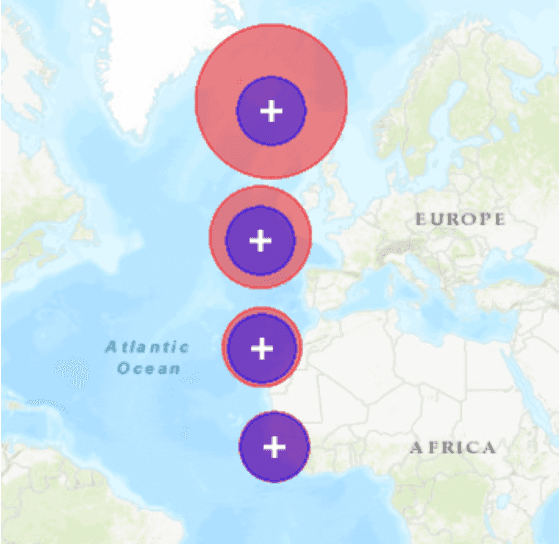## Use location distance measurement analysis in a scene view

`Analysis` and `AnalysisOverlay` classes provide fast and dynamic analysis results in a scene view. The analysis classes allow you to define analyses (such as viewshed, line of sight, and measure) that use data in the current scene and render results dynamically in the view. Analysis results are displayed using an `AnalysisOverlay` in a scene view. For more information about working with analysis overlays, see Manage analysis with analysis overlays.

You can use the `LocationDistanceMeasurement` analysis class to make interactive distance measurements between two locations in the scene view. Because the input locations are 3D points, you can measure between locations on, above, or below the surface.

A location distance measurement returns the following distances:

• Direct distance—The straight-line distance between the start and end locations
• Horizontal distance—The planar distance between the start and end locations as projected onto the surface of the earth
• Vertical distance—The difference in elevation (z-value) between the start and end locations

As you update the measurement end location (perhaps by dragging on the display), the analysis displays a graphic with lines for direct, horizontal, and vertical measurements.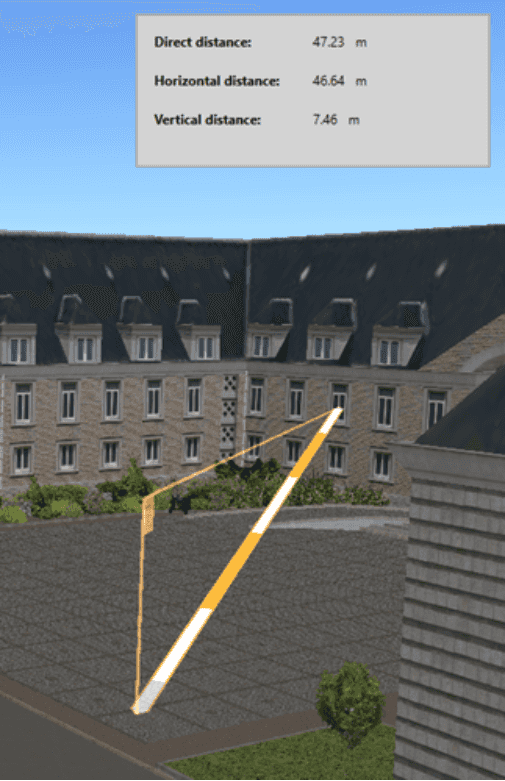Use the following steps to add a location distance measurement analysis to a scene view:

1. Create a new `LocationDistanceMeasurement` and set the start and end locations. These locations must be defined using 3D points (those with x-, y-, and z-coordinate values). To allow the user to define these points interactively, you can define the locations from tap events on the scene view.

Use dark colors for code blocksCopy

``````// Create the scene analysis measure tool and provide the start and end location points.
LocationDistanceMeasurement distanceMeasurement = new LocationDistanceMeasurement(cityCenterPoint,
roofTopPoint);
``````
2. Set the unit system for distance results: metric (meters and kilometers) or imperial (feet and miles).

Use dark colors for code blocksCopy

``````// Set the unit system for the measurements (metric or imperial).
distanceMeasurement.setUnitSystem(UnitSystem.METRIC);
``````
3. Handle the measurement-changed event. When the measurement changes, you can read updated distances. Measurement values will be in the appropriate unit (for the chosen unit system), depending on the length: meters or feet for shorter measurements (under 3,000 meters or 1,000 feet) and kilometers or miles for those that are longer.

Use dark colors for code blocksCopy

``````// Handle the MeasurementChanged event for the measurement analysis.

// Strings to report each distance.
String directDistanceText = "undefined";
String horizontalDistanceText = "undefined";
String verticalDistanceText = "undefined";

// Create a formatted string that shows the direct distance value and units.
if (distanceMeasurement.getDirectDistance() != null) {
double directDistanceValue = distanceMeasurement.getDirectDistance().getValue();
String directDistanceUnit = distanceMeasurement.getDirectDistance().getUnit().getAbbreviation();
directDistanceText = String.format("%.1f %s", directDistanceValue, directDistanceUnit);
}

// Create a formatted string that shows the horizontal distance value and units.
if (distanceMeasurement.getHorizontalDistance() != null) {
double horizontalDistanceValue = distanceMeasurement.getHorizontalDistance().getValue();
String horizontalDistanceUnit = distanceMeasurement.getHorizontalDistance().getUnit().getAbbreviation();
horizontalDistanceText = String.format("%.1f %s", horizontalDistanceValue, horizontalDistanceUnit);
}

// Create a formatted string that shows the vertical distance value and units.
if (distanceMeasurement.getVerticalDistance() != null) {
double verticalDistanceValue = distanceMeasurement.getVerticalDistance().getValue();
String verticalDistanceUnit = distanceMeasurement.getVerticalDistance().getUnit().getAbbreviation();
verticalDistanceText = String.format("%.1f %s", verticalDistanceValue, verticalDistanceUnit);
}

// Show the distances in the UI.
updateDirectDistanceUi(directDistanceText);
updateHorizontalDistanceUi(horizontalDistanceText);
updateVerticalDistanceUi(verticalDistanceText);
});
``````
4. Create an `AnalysisOverlay` with the location distance measurement and add it to the scene view. The distances are not initialized or updated unless the location distance measurement analysis is in an analysis overlay in the scene view's analysis overlay collection.

Use dark colors for code blocksCopy

``````// Create an analysis overlay.
AnalysisOverlay sceneAnalysisOverlay = new AnalysisOverlay();

// Add the LocationDistanceMeasurement to the analysis overlay.